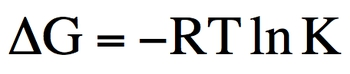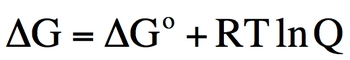## General Chemistry

Learn the toughest concepts covered in Chemistry with step-by-step video tutorials and practice problems by world-class tutors

19. Chemical Thermodynamics

# Gibbs Free Energy

Gibbs Free Energy (ΔG) represents energy associated with a chemical reaction that can be used to do work.

Gibbs Free Energy and Calculations
1
concept

## Gibbs Free Energy6m
Play a video:
2
example

## The reduction of iron (III) oxide with hydrogen produces iron metal and can be written as follows6m
Play a video:

If our Gibbs Free Energy is less than zero then a reaction is spontaneous.

3
Problem

If ∆G is small and positive which of the following statements is true?

If Gibbs Free Energy is less than zero then a reaction will be spontaneous and favor both products and the forward direction.

4
Problem

Nitrogen gas combines with fluorine gas to form nitrogen trifluoride according to the reaction below at 25oC:

N2 (g)  +  3 F2 (g)  →  2 NF3 (g)               ΔHo = -249.0 kJ                       ΔSo = -278 J/K

Calculate ΔGo and state if the reaction favors reactants or products at standard conditions.

To determine if a compound will either freeze or vaporize we must first determine their normal freezing and boiling point by assuming Gibbs Free Energy is zero.

5
example

## For mercury, ΔHvap = 58.5 kJ/mol and ΔSvap = 92.9 J/Kmol at 25°C3m
Play a video:
Hey guys in this new video, we're going to continue with further calculations dealing with Gibbs free energy. So in this example, it says for mercury, the entropy of vaporization equals 58.5 kilojoules per mole. And then the entropy of vaporization equals 92.9 Jews over Kelvin's times moles at 25 degrees Celsius. Now I'm asking you does mercury boil at 3300 and 50 degrees Celsius and one atmosphere pressure. Now this question is similar to something we've seen earlier. What I'm really asking you for is calculating the normal boiling point of mercury. If we can find out the normal boiling point of mercury, we can see if this temperature is high enough for mercury to begin to boil. So remember if we're looking for the normal boiling point of mercury, we're going to assume that delta G zero is equal to zero. And doing this, we're going to say delta G zero equals delta H zero minus T delta S zero. Since this is equal to zero, we can now plug in all, we know we can plug in the delta H value as well as the delta S value So it's similar to something we've seen earlier. So this will be 58.5 kilojoules over moles minus t we don't know temperature. We're looking for it times, remember, enro um entropy of vaporization has to be in kilojoules just like delta H is in kilojoules. So divide that by 1000 gives us 10000.929 kilojoules over K times moles. So we're gonna subtract 58.5 kilojoules over moles. So negative 58.5 kilojoules over moles equals negative temperature times 0.929 kilojoules over K times moles, we need to isolate our temperature. So we're going to divide out negative zero 929 kilojoules over K times moles. OK? So when we do that, this cancels out with this, the negative sign cancels out. We're gonna say that kilojoules and moles will cancel out with these kilojoules and moles leaving us with what units left Kelvin? So temperature here equals 6 29.7 Kelvin. OK. So we're gonna say here that is the normal boiling point of water. So that means the temperature has to be at minimum, this temperature for mercury to begin to boil. So we go back to the 350°C, we have to change it to Kelvin. So add 2, 73.15 we get 6 23.15 Kelvin. And we can see that this temperature here is not high enough. It has to be a minimum of 6:29.7. The temperature we calculated earlier. So we're gonna say no, it does not boil, not hot enough has to be a little bit hotter than that.

Besides our standard equation for Gibbs Free Energy we have another that connects it to our equilibrium constant K6
example

## The chemical reaction, 2 NO2 (g) + Br2 (g), has a ΔS°= 135 J/molK and ΔH°​=926 kJ/mol4m
Play a video:
for this one. It says the chemical reaction to a no to be our gas breaks down to give us to a no to gas plus br to gas. It has an entropy value of 1 35 jewels over moles times K and an entropy value of 9. 26 killed. Jewels over moles, I asked. Calculate the temperature when k q equals 4.50 times 10 to the five. Now, what we need to realize here is where we have to use two equations. I give you Delta s and I give you a Delta H. So what equation connects them together? Delta G zero equals Delta 80 minus t Delta s zero. At the same time, I'm introducing our equilibrium. Constant K. What equation connects, connects delta G decay or that will be Delta G zero equals negative rt Alan Kay. Now we're gonna say that both of these equations are equal to Delta G zero, which would mean that this portion is equal to this portion. So let's make them equal to one another. We're gonna say here Delta H zero minus t Delta s zero equals negative rt lnk. Now, since we're gonna need some room to work this out. I'm gonna remove myself guys from the image so we have more room to work with. So let's plug in what we know. We know what Delta H is. It's 9 26 killer jewels over moles minus temperature, which we don't know here. You need to convert your delta s value in to kill a Jules. So divide this by 1000. So that gives us point 135 killer jewels over most times. K are is your gas constant, which is 8. But actually, we need to convert it toe from that number because it's 8.314 jewels over moles times K. That's what our equals. It's that constant number. But we needed to be in kill jewels because this is in kill jewels as well as this. So we divide that number by 1000 to get this new number temperature. We don't know what temperature is here either. So we just put t again and then Alan Kay, which is 4.50 times 10 to the five. Okay, Now let's combine like terms, so we're gonna multiply this times, Ellen of this answer. So that's gonna give us negative 0.1082 to 3 t Because remember our temperatures still there as a variable and that equals the other side that equals 9 26 minus 260.135 t. We're gonna add 0.135 t to both sides. So 9 26 equals 0.2677 70. We wanna isolate temperature, so divide out the 0. And that will give us a temperature off 3.46 times 10 to the four. Kelvin, Hopefully, you guys were able to follow along when doing this question. And just remember, Delta G zero equals more than just one type of equation here. It equals these two particular types in order to connect them together to find our temperature, you need to know what those equations are in the first place. And after that it would just be simple algebraic methods in order to find our final answer

Under nonstandard conditions the pressure, temperature and molarities will be different from 1.0 atm, 25oC and 1.0 M respectively and so we use the following equation for Gibbs Free Energy:7
example

## Calculate ΔGrxn at 25°C under the conditions shown below for the following reaction2m
Play a video:
Hey, guys, In this new video, we're gonna continue with our discussion on calculations dealing with Gibbs Free Energy. But now look at it under nonstandard conditions. So an example. One, it says. Calculate Delta G of reaction. You'll notice that there isn't a small circle on top, meaning that this is under nonstandard conditions. So calculate Delta G reaction at 25 degrees Celsius under the conditions shown below for the following reaction here I tell you, Delta G, under standard conditions is 31.6 Killer Joel's. Now we know we're dealing with non standard conditions here, so it's gonna be Delta G equals Delta G zero plus r T l N Q. Now what you need to remember is that Q is our reaction quotient and it equals products. Overreact INTs. It ignores solids and liquids. Luckily, neither one of those these compounds is a solid or liquid. So we're gonna look at both of them. So we're gonna take you here equals C l three. The coefficient is a to that. To me, this is going to be the power now divided by l two. It's coefficient is three. So its power will be three is. Well, okay, The partial pressures of seal to unseal three, given as these numbers, respectively. So those are the values were gonna plug in, So C l three is 4.9 squared C l to his 0.83 cubed when we plugged this sin. It gives us 41.9912 So that's what our Q equals. So we're gonna say now Delta G is 31.6 killer jewels plus 0. killer jewels over Calvin times Moles time here will have to be in Kelvin, so add to 73. 15 toe are 25 degrees Celsius. That gives us 2 98 Kelvin and then Allen off 41.9912 when we plug all that in. So we're gonna do Eleanor 41.9912 times to 98.15 times 0. plus 31.6. When you do all that, that gives you 40 0.86 killer jewels. So what we can say about this reaction? It is highly non spontaneous Because remember, if your Delta G is greater than zero, it's a non spontaneous reaction
8
example

## For the reaction4m
Play a video: